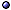Renormalization Theory

In General > s.a. regularization; renormalization group.
* Idea: A procedure for calculating the variation of effective quantities in quantum field theory such as coupling constants and wavefunctions, as the length scales or energy scales change; Can be seen as a way to give a unified description of fundamental and composite/effective degrees of freedom, and understand how they are related; Renormalizability of a theory is seen as guaranteeing its completeness.
* History: Used by Feynman, Schwinger, Tomonaga in QED, by 't Hooft and Veltman for non-abelian gauge theory; Improved by Ken Wilson's work; Dyson's formalism has been superseded by dimensional regularization, especially for gauge theories.
* Motivation: One usually sees the need to renormalize a quantum field theory when one meets the infinities coming up in the calculation of amplitudes for some diagrams, but the concept of renormalization is much more general, and is needed for physical reasons in any interacting theory; For example, the mass of an electron in a crystal is renormalized, as is the mass of a He-filled balloon rising in the air {Carl's class demonstration}.
* Conditions: Renormalizability can be obtained only for s = 0, 1/2, 1.Specific aspects, applications: see critical phenomena; heat [kernel]; mass [classical]; particle models; specific theories.

Approaches, Techniques > s.a. quantum field theory approaches [including avoiding renormalization] and techniques.
* Remark: When one reads that some results in quantum field theory are independent of the regularization method, this really means that the (reasonable) methods that have been tried all give the same result.
* Perturbative renormalization: (i) Expand the quantities of interest in a perturbative series; (ii) Regularize the divergent terms, in order to be able to manipulate them; (iii) Manipulate the free parameters to absorb the regularization parameters in their definitions; If this succeeds, the theory is called (perturbatively) renormalizable, or superrenormalizable, which doesn't necessarily mean that the series we started with will now converge.
* 1-loop: It can be formulated in terms of the heat-kernel expansion.
* Non-perturbative renormalization: Done, e.g., in the lattice approach.
* BPH prescription: (i) Start with a lagrangian density $$\cal L$$ written in terms of renormalized quantities (mass, wave function, coupling constant); (ii) Isolate the divergent parts of the 1PI diagrams by Taylor expansions (around arbitrary points); (iii) Construct a set of counterterms Δ$$\cal L$$(1) to cancel the 1-loop divergences; (iv) Use the new $$\cal L$$ + Δ$$\cal L$$(1) to generate 2-loop diagrams and construct Δ$$\cal L$$(2) to cancel their divergences; (v) Repeat the above steps until the next one does not produce divergences; The resulting lagrangian density is of the form

$$\cal L$$ = $$\cal L$$ + Δ$$\cal L$$ = $$\cal L$$ + ∑i Δ$$\cal L$$(i) .

* Epstein-Glaser approach: A causal perturbation theory framework in which renormalization is equivalent to the extension of time-ordered distributions to coincident points by a modified Taylor subtraction on the corresponding test functions.
* Renormalon: A pattern of divergence of perturbative quantum field theory expansions, related to their small and large momentum behavior.
@ Algebraic: Grassi et al NPB(01)hp; Brennecke & Duetsch a0801-conf [quantum action principle in causal perturbation theory].
@ Epstein-Glaser approach: Epstein & Glaser AIHP(73); Prange JPA(99); Keller JMP(09)-a0902 [euclidean]; Ceyhan a1002 [and Connes-Marcolli renormalization group]; > s.a. renormalization of specific theories [gauge theory, scalar field]; regularization.
@ Other approaches: Egoryan & Manvelyan TMP(86) [stochastic]; Müller & Rau PLB(96)ht/95 [Fock space projectors]; Schnetz JMP(97)ht/96 [differential]; Ni qp/98; Yang ht/98, ht/98-conf; Pernici NPB(00) [dimensional]; 't Hooft IJMPA(05)ht/04-in [without divergences]; Ng & van Dam IJMPA(06)ht/05 [neutrix calculus]; Alexandre ht/05-conf; Gracey ht/06-conf [practicalities]; Costello a0706 [and Batalin-Vilkovisky formalism, on compact manifolds]; Prokhorenko a0707 [non-equilibrium]; Bostelmann et al CMP(09)-a0711 [scaling algebras]; Ebrahimi-Fard & Patras AHP(10)-a1003 [exponential]; Pivovarov a1312 [non-perturbative]; Berghoff CNTP(15)-a1411 [De Concini-Procesi wonderful models]; Todorov NPB(16)-a1611 [causal position space renormalization]; Zucchini JHEP(18)-a1711 [in the Batalin-Vilkovisky theory]; Lang et al CQG(18)-a1711 [Hamiltonian]; Thaler & Vargas Le-Bert a1712 [without series expansions, based on ultrafilters on a projective system of coarse regions]; > s.a. types of theories [asymptotic safety].

References > s.a.  history of quantum physics; Scaling; quantum fields in curved spacetime.
@ Books: Fröhlich 83; Collins 84; Piguet & Sorella 95 [algebraic]; Salmhofer 98 [mathematical introduction]; McComb 03.
@ Reviews, intros: Coleman in(73); Velo & Wightman ed-76; Delamotte AJP(04)feb-ht/02 [intro]; Stefanovich ht/05 [mass and charge]; Collins ht/06-en; Sonoda a0710-ln; Mastropietro 08 [non-perturbative]; Trinchero a1004 [simple example]; Rivasseau a1102 [and interacting Fermi liquids]; Gurău et al a1401 [advanced overview]; Mukhi a1908-ln; Melo a1909-ln.
@ Renormalizability and finiteness: Gegelia & Kiknadze ht/95; Castagnino IJTP(01)qp/00; Bergbauer et al a0908 [resolution of singularities]; Tillman a1009.
@ Coupling-constant renormalization: Elias & Mckeon IJMPA(03) [vanishing of bare in 4D]; Toms PRL(08) [fine-structure constant, cosmological constant contribution]; Rosaler & Harlander a1809; > s.a. gauge-theory renormalization; QCD; quantum-gravity renormalization [for G].
@ Green-function renormalization: Hepp CMP(66) [Bogoliubov-Parasiuk subtraction].
@ Wave-function renormalization: Esposito et al PRD(98) [fermions at finite T].
@ Hopf algebra approach: Connes & Kreimer JHEP(99)ht, CMP(00)ht/99 [and Riemann-Hilbert problem]; Ionescu & Marsalli ht/03 [Hopf algebra deformation]; Ebrahimi-Fard et al PLB(06)ht/05 [combinatorics]; Ebrahimi-Fard & Guo ht/05; Ratsimbarison mp/05 [rev]; Ebrahimi-Fard et al CMP(07) [and Lie algebra]; Panzer a1407-proc [intro]; > s.a. theories [electromagnetism, gauge theory].
@ Mathematical setting: Joglekar JPA(01)ht/00; Vladimirov ht/02 [proofs]; Anselmi PRD(16)-a1511 [cohomology, and the background-field method].
@ Coarse-graining in field theory: Yang ht/00; Calzetta et al PRP(01)ht-proc [semiclassical gravity]; Zapata JPCS(05), Manrique JPCS(05) [without background metric]; Bodendorfer & Haneder a2011 [and lqc].
@ Conceptual: Teller PhSc(89)jun, Huggett & Weingard PhSc(96)sep [and philosophy]; Kadanoff SHPMP(13); Bény & Osborne NJP(15)-a1402 [information in renormalization, and equivalence classes of theories]; Butterfield JPhil-a1406 [reduction and emergence]; Butterfield & Bouatta a1406-in [for philosophers].
@ Related topics: Slavnov TMP(95) [ambiguities], PLB(01) [gauge-invariant]; Kreimer JKTR(97)qa/96-Hab [and knot theory]; Yang ht/00 [constraints on schemes]; Gainutdinov ht/01 [ultraviolet finite non-local effective theory]; Beneke PRP(99) [renormalons]; Polonyi & Sailer PRD(01) [of composite operators]; Thorn ht/03-conf [in string language]; Agarwala LMP(10)-a0909 [geometric formulation]; Caginalp & Esenturk JPA(14) [for higher-order differential equations]; > s.a. Multiscale Physics.